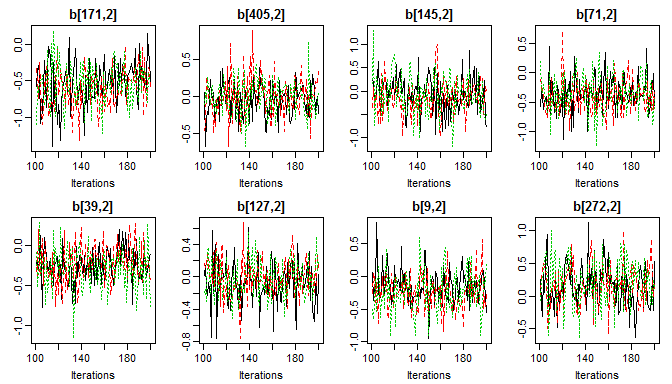# Parameter Selection

### How to specify which parameters to monitor and display in the results.

#### 2018-12-04

In this vignette, we use the NHANES data for examples in cross-sectional data and the dataset simLong for examples in longitudinal data. For more info on these datasets, check out the vignette Visualizing Incomplete Data, in which the distribution of variables and missing values in both sets is explored.

Note:
In many of the examples we use n.adapt = 0 (and n.iter = 0, which is the default) in order to prevent the MCMC sampling to reduce computational some time. mess = FALSE is used to suppress messages that are not of interest in this vignette. progress.bar = 'none' prevents printing of the progress of the MCMC sampling, which results in lengthy output in the vignette, but is useful in practice.

## Monitoring parameters

JointAI uses JAGS for performing the MCMC (Markov Chain Monte Carlo) sampling. Since JAGS only saves the values of MCMC chains for those parameters/variables for which the user has specified that they should be monitored, this is also the case in JointAI.

For this purpose, lm_imp(), glm_imp(), lme_imp(), glme_imp() and survreg_imp() have an argument monitor_params.

monitor_params takes a named list (often a named vector also works) with the following possible entries:

name/key word what is monitored
analysis_main betas, tau_y and sigma_y
analysis_random ranef, D, invD, RinvD
imp_pars alphas, tau_imp, gamma_imp, delta_imp
imps imputed values
betas regression coefficients of the analysis model
tau_y precision of the residuals from the analysis model
sigma_y standard deviation of the residuals from the analysis model
ranef random effects
D covariance matrix of the random effects
invD inverse of D
RinvD scale matrix in Wishart prior for invD
alphas regression coefficients in the imputation models
tau_imp precision parameters of the residuals from imputation models
gamma_imp intercepts in ordinal imputation models
delta_imp increments of ordinal intercepts
other additional parameters

Each of the key words works as a switch.

### Parameters of the analysis model

The default setting is monitor_params = c(analysis_main = TRUE), i.e., only the main parameters of the analysis model are monitored, and monitoring is switched off for all other parameters.

The main parameters are the regression coefficients of the analysis model (beta) and the residual standard deviation (sigma_y, and precision tau_y).

The function parameters() returns the parameters that are specified to be followed (even for models where no MCMC sampling was performed, i.e. when n.iter = 0 and n.adapt = 0).

For example:

lm1 <- lm_imp(SBP ~ gender + WC + alc + creat, data = NHANES,
n.adpat = 0, mess = FALSE)

parameters(lm1, mess = FALSE)
#>  "(Intercept)"  "genderfemale" "WC"           "creat"
#>  "alc>=1"       "sigma_SBP"

### Imputed values & parameters of the imputation models

To generate (multiple) imputed datasets that can be used for further analyses, the imputed values need to be monitored. This can be done by setting monitor_params = c(imps = TRUE).

lm2 <- lm_imp(SBP ~ age + WC + alc + smoke + occup,
data = NHANES, n.adapt = 0, mess = FALSE,
monitor_params = c(imps = TRUE, analysis_main = FALSE)
)

parameters(lm2, mess = FALSE)
#>   "Xc[33,3]"    "Xc[150,3]"   "Xc[1,8]"     "Xc[7,8]"     "Xc[8,8]"
#>   "Xc[12,8]"    "Xc[13,8]"    "Xc[21,8]"    "Xc[22,8]"    "Xc[31,8]"
#>  "Xc[33,8]"    "Xc[34,8]"    "Xc[39,8]"    "Xc[49,8]"    "Xc[66,8]"
#>  "Xc[67,8]"    "Xc[80,8]"    "Xc[86,8]"    "Xc[91,8]"    "Xc[92,8]"
#>  "Xc[105,8]"   "Xc[111,8]"   "Xc[115,8]"   "Xc[118,8]"   "Xc[120,8]"
#>  "Xc[127,8]"   "Xc[132,8]"   "Xc[139,8]"   "Xc[146,8]"   "Xc[149,8]"
#>  "Xc[152,8]"   "Xc[163,8]"   "Xc[165,8]"   "Xc[169,8]"   "Xc[180,8]"
#>  "Xc[185,8]"   "Xcat[6,1]"   "Xcat[16,1]"  "Xcat[24,1]"  "Xcat[27,1]"
#>  "Xcat[45,1]"  "Xcat[57,1]"  "Xcat[58,1]"  "Xcat[60,1]"  "Xcat[61,1]"
#>  "Xcat[65,1]"  "Xcat[66,1]"  "Xcat[70,1]"  "Xcat[80,1]"  "Xcat[81,1]"
#>  "Xcat[85,1]"  "Xcat[88,1]"  "Xcat[89,1]"  "Xcat[90,1]"  "Xcat[101,1]"
#>  "Xcat[104,1]" "Xcat[116,1]" "Xcat[133,1]" "Xcat[137,1]" "Xcat[143,1]"
#>  "Xcat[168,1]" "Xcat[170,1]" "Xcat[180,1]" "Xcat[186,1]" "Xcat[16,2]"
#>  "Xcat[99,2]"  "Xcat[123,2]" "Xcat[156,2]" "Xcat[158,2]" "Xcat[166,2]"
#>  "Xcat[172,2]"

JointAI uses a number of different design matrices to store different types of variables. The matrix Xc is the design matrix of cross-sectional covariates. For categorical incomplete variables (with more than 2 categories) the original variable is stored in the matrix Xcat and Xc contains the corresponding dummy coded variables. Hence, the imputed values of continuous and binary variables are elements of Xc and imputed values of categorical variables are elements of Xcat.

The parameters of the models for the incomplete variables can be selected with monitor_params = c(imp_pars = TRUE). This will set monitors for the regression coefficients (alpha) and other parameters, such as precision (tau_*) and intercepts & increments (gamma_* and delta_*) in cumulative logit models.

lm3 <- lm_imp(SBP ~ age + WC + alc + smoke + occup,
data = NHANES, n.adapt = 0, mess = FALSE,
monitor_params = c(imp_pars = TRUE, analysis_main = FALSE),
)

parameters(lm3, mess = FALSE)
#>  "alpha"       "tau_WC"      "gamma_smoke" "delta_smoke"

#### Side note: Getting information about of the imputation models

An overview of the imputation models used, including the names of the parameters and the hyperparameters can be obtained with

list_impmodels(lm2)
#> Normal imputation model for 'WC'
#> * Predictor variables:
#>   (Intercept), age
#> * Regression coefficients:
#>   alpha[1:2] (normal prior(s) with mean 0 and precision 1e-04)
#> * Pecision of 'WC':
#>   tau_WC (Gamma prior with scale parameter 0.01 and rate parameter 0.01)
#>
#> Cumulative logit imputation model for 'smoke'
#> * Reference category: 'never'
#> * Predictor variables:
#>   age, WC
#> * Regression coefficients (without intercept):
#>   alpha[3:4] (normal prior(s) with mean 0 and precision 0.001)
#> * Intercepts:
#>   - never: gamma_smoke (normal prior with mean 0 and precision 0.001)
#>   - former: gamma_smoke = gamma_smoke + exp(delta_smoke)
#> * Increments:
#>   delta_smoke (normal prior(s) with mean 0 and precision 0.001)
#>
#> Multinomial logit imputation model for 'occup'
#> * Reference category: 'working'
#> * Predictor variables:
#>   (Intercept), age, WC, smokeformer, smokecurrent
#> * Regression coefficients:
#>   - 'occuplooking for work': alpha[5:9] (normal prior(s) with mean 0 and precision 0.001)
#>   - 'occupnot working': alpha[10:14] (normal prior(s) with mean 0 and precision 0.001)
#>
#> Logistic imputation model for 'alc'
#> * Reference category: '<1'
#> * Predictor variables:
#>   (Intercept), age, WC, smokeformer, smokecurrent, occuplooking for work, occupnot working
#> * Regression coefficients:
#>   alpha[15:21] (normal prior(s) with mean 0 and precision 0.001)

#### Side note: How to extract imputed datasets

Imputed datasets can be extracted and exported with the function get_MIdat(). A completed dataset is created by taking the imputed values from a randomly chosen iteration of the MCMC sample (transforming them back to the original scale, if scaling had been performed during the MCMC sampling) and filling them into the original, incomplete data.

get_MIdat() returns a long-format data.frame containing the imputed datasets (and possibly the original data) stacked onto each other. The imputation number is given in the variable Imputation_, column .id contains a newly created id variable for each observation in cross-sectional data (multi-level data should already contain an id variable).

get_MIdat() takes the arguments:

argument explanation
object a JointAI object
m number of datasets to be created
include logical; should the original data be included?
start the first iteration that may be randomly chosen (i.e., all previous iterations are discarded as burn-in)
minspace minimum number of iterations between iterations chosen as imputed values
seed optional seed value in order to make the random selection
of iterations reproducible
export_to_SPSS logical; should the datasets be exported to SPSS, i.e., written as .txt and .sps file? If export_to_SPSS = FALSE (default) the imputed data is only returned data.frame
resdir directory the files are exported to
filename the name of the .txt and .sps files

### Random effects

For mixed models, analysis_main also includes the random effects covariance matrix D:

lme1 <- lme_imp(bmi ~ age + EDUC, random = ~age | ID,
data = simLong, n.adapt = 0, mess = FALSE)

parameters(lme1, mess = FALSE)
#>  "(Intercept)" "EDUCmid"     "EDUClow"     "age"         "sigma_bmi"
#>  "D[1,1]"      "D[1,2]"      "D[2,2]"

Setting analysis_random = TRUE will switch on monitoring for the random effects (ranef), random effects covariance matrix (D), inverse of the random effects covariance matrix (invD) and the diagonal of the scale matrix of the Wishart-prior of invD (RinvD).

lme2 <- lme_imp(bmi ~ age + EDUC, random = ~age | ID,
data = simLong, n.adapt = 0, mess = FALSE,
monitor_params = c(analysis_random = TRUE))

parameters(lme2, mess = FALSE)
#>   "(Intercept)" "EDUCmid"     "EDUClow"     "age"         "sigma_bmi"
#>   "b"           "invD[1,1]"   "invD[1,2]"   "invD[2,2]"   "D[1,1]"
#>  "D[1,2]"      "D[2,2]"      "RinvD[1,1]"  "RinvD[2,2]"

It is possible to select only a subset of the random effects parameters by specifying them directly, e.g.

lme3a <- lme_imp(bmi ~ age + EDUC, random = ~age | ID,
data = simLong, n.adapt = 0, mess = FALSE,
monitor_params = c(analysis_main = TRUE, RinvD = TRUE))

parameters(lme3a, mess = FALSE)
#>   "(Intercept)" "EDUCmid"     "EDUClow"     "age"         "sigma_bmi"
#>   "D[1,1]"      "D[1,2]"      "D[2,2]"      "RinvD[1,1]"  "RinvD[2,2]"

or by switching unwanted parts of analysis_random off, e.g.

lme3b <- lme_imp(bmi ~ age + EDUC, random = ~age | ID,
data = simLong, n.adapt = 0, mess = FALSE,
monitor_params = c(analysis_main = TRUE,
analysis_random = TRUE,
RinvD = FALSE,
ranef = FALSE))

parameters(lme3b, mess = FALSE)
#>   "(Intercept)" "EDUCmid"     "EDUClow"     "age"         "sigma_bmi"
#>   "invD[1,1]"   "invD[1,2]"   "invD[2,2]"   "D[1,1]"      "D[1,2]"
#>  "D[2,2]"

### Other parameters

The element other in monitor_params allows to specify one or multiple parameters additional parameters to be monitored. When other is used with more than one element, monitor_params has to be a list.

Here, we monitor the probability to be in the alc>=1 group for subjects 1 through 3 and the expected value of the distribution of creat for the first subject.

lm4 <- lm_imp(SBP ~ gender + WC + alc + creat,
data = NHANES, n.adapt = 0, mess = FALSE, progress.bar = 'none',
monitor_params = list(analysis_main = FALSE,
other = c('p_alc[1:3]', "mu_creat")))

parameters(lm4)
#> 'lm4' does not contain MCMC samples.
#>  "p_alc[1:3]"  "mu_creat"

## Subsets of Parameters for Plots, Summaries, etc.

The functions summary(), traceplot(), densplot(), GR_crit() and MC_error() all have an argument subset. This argument allows to select a subset of parameters to be shown in the output. Especially when not only the parameters of the main analysis model are followed, but also, for example, imputed values, looking at a subset may be desirable.

subset follows the same logic as monitor_params described above.

By default, only the parameters of the main analysis model are displayed if they were monitored:

# Run a model monitoring analysis parameters and imputation parameters
lm5 <- lm_imp(SBP ~ gender + WC + alc + creat,
data = NHANES, n.iter = 100, mess = FALSE, progress.bar = 'none',
monitor_params = c(imp_pars = TRUE))

# model summary
summary(lm5)
#>
#>  Linear model fitted with JointAI
#>
#> Call:
#> lm_imp(formula = SBP ~ gender + WC + alc + creat, data = NHANES,
#>     n.iter = 100, monitor_params = c(imp_pars = TRUE), progress.bar = "none",
#>     mess = FALSE)
#>
#> Posterior summary:
#>                Mean    SD   2.5%   97.5% tail-prob. GR-crit
#> (Intercept)  82.209 9.860 62.383 100.764     0.0000   0.996
#> genderfemale  0.430 2.670 -4.135   5.658     0.9200   1.056
#> WC            0.298 0.073  0.153   0.428     0.0000   1.028
#> creat         7.341 7.377 -6.298  20.069     0.3133   1.039
#> alc>=1        6.217 2.605  0.662  11.254     0.0333   1.021
#>
#> Posterior summary of residual std. deviation:
#>           Mean    SD 2.5% 97.5% GR-crit
#> sigma_SBP 14.4 0.755   13  15.8    1.03
#>
#>
#> MCMC settings:
#> Iterations = 101:200
#> Sample size per chain = 100
#> Thinning interval = 1
#> Number of chains = 3
#>
#> Number of observations: 186

# traceplot of the MCMC sample
traceplot(lm5)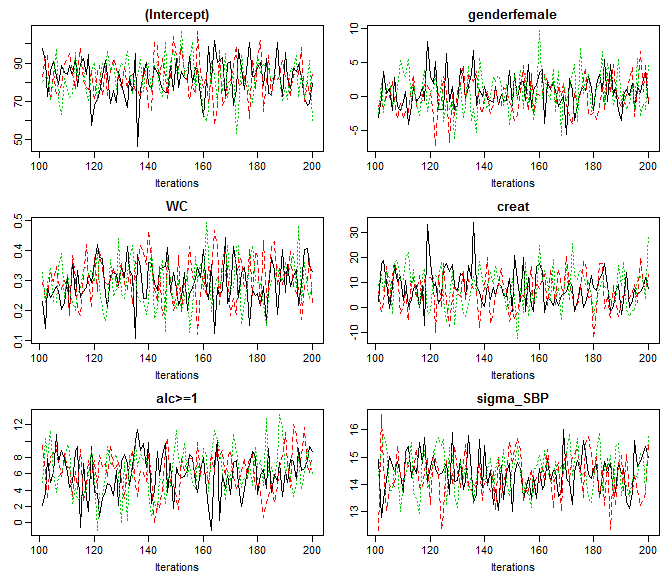# density plot of the MCMC sample
densplot(lm5)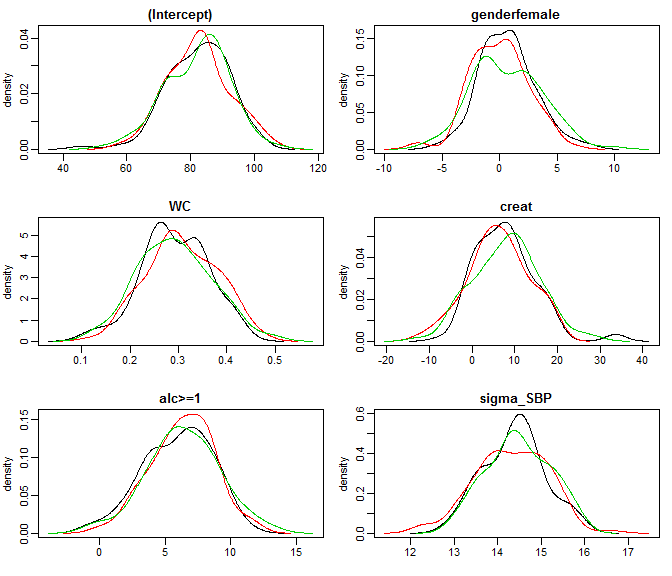# Gelman-Rubin criterion
GR_crit(lm5)
#> Potential scale reduction factors:
#>
#>              Point est. Upper C.I.
#> (Intercept)       0.995      0.996
#> genderfemale      1.018      1.056
#> WC                1.005      1.028
#> creat             1.008      1.039
#> alc>=1            1.005      1.021
#> sigma_SBP         1.007      1.028
#>
#> Multivariate psrf
#>
#> 1.03

# Monte Carlo Error of the MCMC sample
MC_error(lm5)
#>                est    MCSE     SD MCSE/SD
#> (Intercept)  44.09 2.81434 43.614   0.065
#> genderfemale  0.43 0.18131  2.670   0.068
#> WC            0.02 0.00036  0.005   0.071
#> creat        42.88 2.66867 43.092   0.062
#> alc>=1        6.22 0.24649  2.605   0.095
#> sigma_SBP    14.39 0.03915  0.755   0.052

When analysis_main was not switched on, all parameters are displayed by default:

# Re-run the model from above, now creating MCMC samples
lm4 <- lm_imp(SBP ~ gender + WC + alc + creat,
data = NHANES, n.iter = 100, mess = FALSE,  progress.bar = 'none',
monitor_params = list(analysis_main = FALSE,
other = c('p_alc[1:3]', "mu_creat")))

traceplot(lm4, ncol = 4)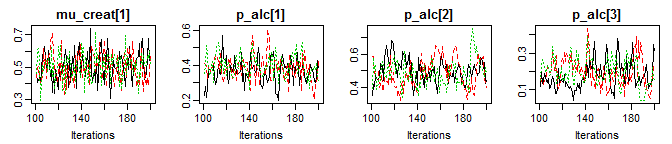### Select a subset of the variables to display

To display other parts of the MCMC sample, subset needs to be specified:

# we use lm5 from above
GR_crit(lm5, subset = c(analysis_main = FALSE, imp_pars = TRUE))
#> Potential scale reduction factors:
#>
#>           Point est. Upper C.I.
#> alpha       0.997      0.998
#> alpha       1.006      1.022
#> alpha       0.997      0.998
#> alpha       0.999      1.008
#> alpha       1.020      1.069
#> alpha       1.007      1.016
#> alpha       0.999      1.008
#> alpha       0.999      1.003
#> alpha       0.999      1.002
#> tau_WC         1.004      1.027
#> tau_creat      1.008      1.029
#>
#> Multivariate psrf
#>
#> 1.03

To select only some of the parameters, they can be specified directly by name via the other element of subset:

summary(lm5, subset = list(other = c('creat', 'alc>=1')))
#>
#>  Linear model fitted with JointAI
#>
#> Call:
#> lm_imp(formula = SBP ~ gender + WC + alc + creat, data = NHANES,
#>     n.iter = 100, monitor_params = c(imp_pars = TRUE), progress.bar = "none",
#>     mess = FALSE)
#>
#> Posterior summary:
#>        Mean   SD   2.5% 97.5% tail-prob. GR-crit
#> creat  7.34 7.38 -6.298  20.1     0.3133    1.04
#> alc>=1 6.22 2.60  0.662  11.3     0.0333    1.02
#>
#>
#> MCMC settings:
#> Iterations = 101:200
#> Sample size per chain = 100
#> Thinning interval = 1
#> Number of chains = 3
#>
#> Number of observations: 186

This also works when a subset of the imputed values should be displayed:

# Re-run the model from above, now creating MCMC samples
lm2 <- lm_imp(SBP ~ age + WC + alc + smoke + occup,
data = NHANES, n.iter = 100, mess = FALSE,  progress.bar = 'none',
monitor_params = c(imps = TRUE, analysis_main = FALSE),
)

# select only imputed values for 'WC' (3rd column of Xc)
sub3 <- grep('Xc\$[[:digit:]]+,3\$', parameters(lm2), value = TRUE)
sub3
#>  "Xc[33,3]"  "Xc[150,3]"

traceplot(lm2, subset = list(other = sub3), ncol = 2)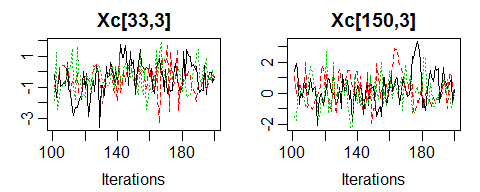### Random subset of subject-specific values

When the number of imputed values is larger, or in order to check convergence of random effects, it may not be feasible to plot all traceplots. In that case, a random subset of, for instance the random effects, can be selected:

lme4 <- lme_imp(bmi ~ age + EDUC, random = ~age|ID,
data = simLong, n.iter = 100, mess = FALSE, progress.bar = 'none',
monitor_params = c(analysis_main = FALSE, ranef = TRUE))

# exract random intercepts
ri <- grep('^b\$[[:digit:]]+,1\$$', colnames(lme4$MCMC[]), value = T)

# extract random slopes
rs <- grep('^b\$[[:digit:]]+,2\$$', colnames(lme4$MCMC[]), value = T)

# plot the chains of 8 randomly selected random intercepts
traceplot(lme4, subset = list(other = sample(ri, size = 8)), ncol = 4)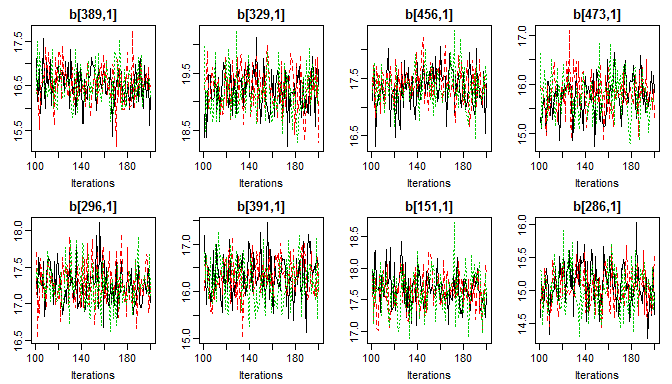# plot the chains of 8 randomly selected random slopes
traceplot(lme4, subset = list(other = sample(rs, size = 8)), ncol = 4)